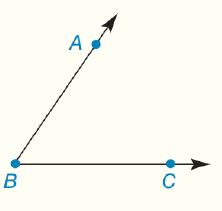Chapter 1.CT, Problem 2CT### Elementary Geometry for College St...

6th Edition
Daniel C. Alexander + 1 other
ISBN: 9781285195698

#### Solutions

Chapter
Section### Elementary Geometry for College St...

6th Edition
Daniel C. Alexander + 1 other
ISBN: 9781285195698
Textbook Problem
139 views

# Given ∠ A B C (as shown), provide a second correct method for naming this angle.To determine

To find:

The second correct method for naming the given angle.

Explanation

Given:

The given figure is,

Approach:

To name an angle correctly, use three point letters. The second letter must be the vertex and the first and third letter must be a point on the rays that are the sides of the angle. So, for ABC, B is the vertex and A is the point at ray

### Still sussing out bartleby?

Check out a sample textbook solution.

See a sample solution

#### The Solution to Your Study Problems

Bartleby provides explanations to thousands of textbook problems written by our experts, many with advanced degrees!

Get Started

#### 2. Find all first and second partial derivatives of

Mathematical Applications for the Management, Life, and Social Sciences

#### f(x)=1x2+1; limxf(x); and limxf(x) x 1 10 100 1000 f(x) x 1 10 100 1000 f(x)

Applied Calculus for the Managerial, Life, and Social Sciences: A Brief Approach

#### Evaluate the integral. 18x2/3dx

Single Variable Calculus: Early Transcendentals

#### Given that for all x, a power series for is:

Study Guide for Stewart's Single Variable Calculus: Early Transcendentals, 8th